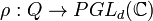# Criterion for projective representation to lift to linear representation

## Statement

Suppose$G$ is a finite group and$N$ is a central subgroup of$G$. Suppose$Q = G/N$ and$\rho: Q \to PGL_d(\mathbb{C})$ is a homomorphism of groups, and hence a projective representation of$G/N$. Let$\alpha:G \to Q$ and$\pi_d:GL_d(\mathbb{C}) \to PGL_d(\mathbb{C})$ be the obvious quotient maps. We call a linear representation$\theta: G \to GL_d(\mathbb{C})$ a lift of$\rho$ if$\pi_d \circ \theta = \rho \circ \alpha$.

The statement is that there exists a linear representation that is a lift of$\rho$ if and only if the following holds.

### Description of condition in cohomology language$H^2(Q;N) \times \operatorname{Hom}(N,\mathbb{C}^*) \to H^2(Q;\mathbb{C}^*)$

Fixing the element of$H^2(Q;N)$ corresponding to the extension group$G$, we get a homomorphism:$\operatorname{Hom}(N,\mathbb{C}^*) \to H^2(Q;\mathbb{C}^*)$

from the group of one-dimensional representations of the central subgroup$N$ to$H^2(Q;\mathbb{C}^*)$.

The projective representation$\rho$ lifts to a linear representation if and only if the image of the above homomorphism contains the cohomology class (i.e., the element of$H^2(Q;\mathbb{C}^*)$ corresponding to$\rho$.

### Description of condition in homology language$0 \to \operatorname{Ext}^1(Q^{\operatorname{ab}},N) \to H^2(Q;N) \to \operatorname{Hom}(H_2(Q;\mathbb{Z}),N) \to 0$

The extension group$G$ corresponds to an element of$H^2(Q;N)$, which hence maps to an element of$\operatorname{Hom}(H_2(Q;\mathbb{Z}),N)$. By composition, this defines a map:$\operatorname{Hom}(N,\mathbb{C}^*) \to \operatorname{Hom}(H_2(Q;\mathbb{Z}),\mathbb{C}^*) = H^2(Q;\mathbb{C}^*)$

The condition is that the image of this homomorphism should contain the cohomology class corresponding to the projective representation.

## Particular cases

Case Conclusion
the central subgroup is trivial. In this case, a projective representation lifts to a linear representation if and only if its cohomology class is trivia.$G$ is a Schur covering group of$Q$. In this case, every projective representation of$Q$ lifts to a linear representation of$G$.